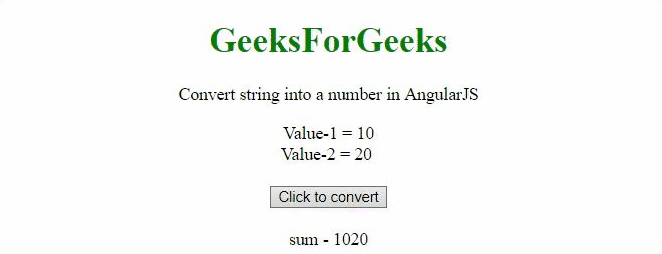# How to convert string into a number using AngularJS ?

In this article, we will see how to convert a string into a number with the help of AngularJS.

Approach:

• The parseInt() method is used for converting the string to an integer.
• We will check whether the string is an integer or not by the isNumber() method.

Example 1: In the first example, the string ’90’ is converted to an integer.

## HTML

 ` ` `<``html``> ` ` `  `<``head``> ` `    ``<``script` `src``= ` `"https://ajax.googleapis.com/ajax/libs/angularjs/1.2.13/angular.min.js"``> ` `    `` ` ` `  `    ``<``script``> ` `        ``var myApp = angular.module("app", []); ` `        ``myApp.controller("controller", function (\$scope) { ` `            ``\$scope.a = '90'; ` `            ``\$scope.isNumberA = angular.isNumber(\$scope.a); ` `            ``\$scope.convertToInt = function () { ` `                ``\$scope.a = parseInt(\$scope.a); ` `                ``\$scope.isNumberA = angular.isNumber(\$scope.a); ` `            ``}; ` `        ``}); ` `    `` ` ` ` ` `  `<``body` `style``=``"text-align:center;"``> ` `    ``<``h1` `style``=``"color:green;"``> ` `        ``GeeksForGeeks ` `    `` ` ` `  ` `  `    ``<``p``> ` `        ``Convert string into a  ` `        ``number in AngularJS ` `    `` ` ` `  ` `  `    ``<``div` `ng-app``=``"app"``> ` `        ``<``div` `ng-controller``=``"controller"``> ` `            ``Value = {{a}} ` `            ``<``br``> ` `            ``<``br``> ` `            ``<``button` `ng-click``=``'convertToInt()'``> ` `                ``Click to convert ` `            `` ` `            ``<``br``> ` `            ``<``br``> ` `            ``isNumber(value) - {{isNumberA}}<``br``> ` `        `` ` `    `` ` ` ` ` `  ` `

Output:Example 2: The 2 strings are added and then converted to an integer and then added again.

## HTML

 ` ` `<``html``> ` ` `  `<``head``> ` `    ``<``script` `src``= ` `"https://ajax.googleapis.com/ajax/libs/angularjs/1.2.13/angular.min.js"``> ` `    `` ` ` `  `    ``<``script``> ` `        ``var myApp = angular.module("app", []); ` `        ``myApp.controller("controller", function (\$scope) { ` `            ``\$scope.a = "10"; ` `            ``\$scope.b = "20"; ` `            ``\$scope.strToInt = function () { ` `                ``\$scope.a = parseInt(\$scope.a); ` `                ``\$scope.b = parseInt(\$scope.b); ` `            ``}; ` `        ``}); ` `    `` ` ` ` ` `  `<``body` `style``=``"text-align:center;"``> ` `    ``<``h1` `style``=``"color:green;"``> ` `        ``GeeksForGeeks ` `    `` ` ` `  `    ``<``p``> ` `        ``Convert string into a  ` `        ``number in AngularJS ` `    `` ` ` `  ` `  `    ``<``div` `ng-app``=``"app"``> ` `        ``<``div` `ng-controller``=``"controller"``> ` `            ``Value-1 = {{a}} ` `            ``<``br``> ` `            ``Value-2 = {{b}} ` `            ``<``br``><``br``> ` `            ``<``button` `ng-click``=``'strToInt()'``> ` `                ``Click to convert ` `            `` ` `            ``<``br``><``br``> ` `            ``sum - {{a + b}}<``br``> ` `        `` ` `    `` ` ` ` ` `  ` `

Output:My Personal Notes arrow_drop_upCheck out this Author's contributed articles.

If you like GeeksforGeeks and would like to contribute, you can also write an article using contribute.geeksforgeeks.org or mail your article to contribute@geeksforgeeks.org. See your article appearing on the GeeksforGeeks main page and help other Geeks.

Please Improve this article if you find anything incorrect by clicking on the "Improve Article" button below.

Article Tags :
Practice Tags :

Be the First to upvote.

Please write to us at contribute@geeksforgeeks.org to report any issue with the above content.